$$\require{cancel}$$

# 7.E: Applications of Newton's Laws (Exercises)

•• Contributed by OpenStax
• General Physics at OpenStax CNX

## Conceptual Questions

### 6.1 Solving Problems with Newton’s Laws

1. To simulate the apparent weightlessness of space orbit, astronauts are trained in the hold of a cargo aircraft that is accelerating downward at g. Why do they appear to be weightless, as measured by standing on a bathroom scale, in this accelerated frame of reference? Is there any difference between their apparent weightlessness in orbit and in the aircraft?

### 6.2 Friction

1. The glue on a piece of tape can exert forces. Can these forces be a type of simple friction? Explain, considering especially that tape can stick to vertical walls and even to ceilings.
2. When you learn to drive, you discover that you need to let up slightly on the brake pedal as you come to a stop or the car will stop with a jerk. Explain this in terms of the relationship between static and kinetic friction.
3. When you push a piece of chalk across a chalkboard, it sometimes screeches because it rapidly alternates between slipping and sticking to the board. Describe this process in more detail, in particular, explaining how it is related to the fact that kinetic friction is less than static friction. (The same slip-grab process occurs when tires screech on pavement.)
4. A physics major is cooking breakfast when she notices that the frictional force between her steel spatula and Teflon frying pan is only 0.200 N. Knowing the coefficient of kinetic friction between the two materials, she quickly calculates the normal force. What is it?

### 6.3 Centripetal Force

1. If you wish to reduce the stress (which is related to centripetal force) on high-speed tires, would you use large- or small-diameter tires? Explain.
2. Define centripetal force. Can any type of force (for example, tension, gravitational force, friction, and so on) be a centripetal force? Can any combination of forces be a centripetal force?
3. If centripetal force is directed toward the center, why do you feel that you are ‘thrown’ away from the center as a car goes around a curve? Explain.
4. Race car drivers routinely cut corners, as shown below (Path 2). Explain how this allows the curve to be taken at the greatest speed.1. Many amusement parks have rides that make vertical loops like the one shown below. For safety, the cars are attached to the rails in such a way that they cannot fall off. If the car goes over the top at just the right speed, gravity alone will supply the centripetal force. What other force acts and what is its direction if:
1. The car goes over the top at faster than this speed?
2. The car goes over the top at slower than this speed?1. What causes water to be removed from clothes in a spin-dryer?
2. As a skater forms a circle, what force is responsible for making his turn? Use a free-body diagram in your answer.
3. Suppose a child is riding on a merry-go-round at a distance about halfway between its center and edge. She has a lunch box resting on wax paper, so that there is very little friction between it and the merry-go-round. Which path shown below will the lunch box take when she lets go? The lunch box leaves a trail in the dust on the merry-go-round. Is that trail straight, curved to the left, or curved to the right? Explain your answer.1. Do you feel yourself thrown to either side when you negotiate a curve that is ideally banked for your car’s speed? What is the direction of the force exerted on you by the car seat?
2. Suppose a mass is moving in a circular path on a frictionless table as shown below. In Earth’s frame of reference, there is no centrifugal force pulling the mass away from the center of rotation, yet there is a force stretching the string attaching the mass to the nail. Using concepts related to centripetal force and Newton’s third law, explain what force stretches the string, identifying its physical origin.1. When a toilet is flushed or a sink is drained, the water (and other material) begins to rotate about the drain on the way down. Assuming no initial rotation and a flow initially directly straight toward the drain, explain what causes the rotation and which direction it has in the Northern Hemisphere. (Note that this is a small effect and in most toilets the rotation is caused by directional water jets.) Would the direction of rotation reverse if water were forced up the drain?
2. A car rounds a curve and encounters a patch of ice with a very low coefficient of kinetic fiction. The car slides off the road. Describe the path of the car as it leaves the road.
3. In one amusement park ride, riders enter a large vertical barrel and stand against the wall on its horizontal floor. The barrel is spun up and the floor drops away. Riders feel as if they are pinned to the wall by a force something like the gravitational force. This is an inertial force sensed and used by the riders to explain events in the rotating frame of reference of the barrel. Explain in an inertial frame of reference (Earth is nearly one) what pins the riders to the wall, and identify all forces acting on them.
4. Two friends are having a conversation. Anna says a satellite in orbit is in free fall because the satellite keeps falling toward Earth. Tom says a satellite in orbit is not in free fall because the acceleration due to gravity is not 9.80 m/s2. Who do you agree with and why?
5. A nonrotating frame of reference placed at the center of the Sun is very nearly an inertial one. Why is it not exactly an inertial frame?

### 6.4 Drag Force and Terminal Speed

1. Athletes such as swimmers and bicyclists wear body suits in competition. Formulate a list of pros and cons of such suits.
2. Two expressions were used for the drag force experienced by a moving object in a liquid. One depended upon the speed, while the other was proportional to the square of the speed. In which types of motion would each of these expressions be more applicable than the other one?
3. As cars travel, oil and gasoline leaks onto the road surface. If a light rain falls, what does this do to the control of the car? Does a heavy rain make any difference?
4. Why can a squirrel jump from a tree branch to the ground and run away undamaged, while a human could break a bone in such a fall?

## Problems

### 6.1 Solving Problems with Newton’s Laws

1. A 30.0-kg girl in a swing is pushed to one side and held at rest by a horizontal force $$\vec{F}$$ so that the swing ropes are 30.0° with respect to the vertical. (a) Calculate the tension in each of the two ropes supporting the swing under these conditions. (b) Calculate the magnitude of $$\vec{F}$$.
2. Find the tension in each of the three cables supporting the traffic light if it weighs 2.00 x 102 N.1. Three forces act on an object, considered to be a particle, which moves with constant velocity v = (3 $$\hat{i}$$ − 2 $$\hat{j}$$) m/s. Two of the forces are $$\vec{F}_{1}$$ = (3 $$\hat{i}$$ + 5 $$\hat{j}$$ − 6k $$\hat{k}$$) N and $$\vec{F}_{2}$$ = (4 $$\hat{i}$$ − 7 $$\hat{j}$$ + 2 $$\hat{k}$$) N. Find the third force.
2. A flea jumps by exerting a force of 1.20 x 10−5 N straight down on the ground. A breeze blowing on the flea parallel to the ground exerts a force of 0.500 x 10−6 N on the flea while the flea is still in contact with the ground. Find the direction and magnitude of the acceleration of the flea if its mass is 6.00 x 10−7 kg . Do not neglect the gravitational force.
3. Two muscles in the back of the leg pull upward on the Achilles tendon, as shown below. (These muscles are called the medial and lateral heads of the gastrocnemius muscle.) Find the magnitude and direction of the total force on the Achilles tendon. What type of movement could be caused by this force?1. After a mishap, a 76.0-kg circus performer clings to a trapeze, which is being pulled to the side by another circus artist, as shown here. Calculate the tension in the two ropes if the person is momentarily motionless. Include a free-body diagram in your solution.1. A 35.0-kg dolphin decelerates from 12.0 to 7.50 m/s in 2.30 s to join another dolphin in play. What average force was exerted to slow the first dolphin if it was moving horizontally? (The gravitational force is balanced by the buoyant force of the water.)
2. When starting a foot race, a 70.0-kg sprinter exerts an average force of 650 N backward on the ground for 0.800 s. (a) What is his final speed? (b) How far does he travel?
3. A large rocket has a mass of 2.00 x 106 kg at takeoff, and its engines produce a thrust of 3.50 x 107 N. (a) Find its initial acceleration if it takes off vertically. (b) How long does it take to reach a velocity of 120 km/h straight up, assuming constant mass and thrust?
4. A basketball player jumps straight up for a ball. To do this, he lowers his body 0.300 m and then accelerates through this distance by forcefully straightening his legs. This player leaves the floor with a vertical velocity sufficient to carry him 0.900 m above the floor. (a) Calculate his velocity when he leaves the floor. (b) Calculate his acceleration while he is straightening his legs. He goes from zero to the velocity found in (a) in a distance of 0.300 m. (c) Calculate the force he exerts on the floor to do this, given that his mass is 110.0 kg.
5. A 2.50-kg fireworks shell is fired straight up from a mortar and reaches a height of 110.0 m. (a) Neglecting air resistance (a poor assumption, but we will make it for this example), calculate the shell’s velocity when it leaves the mortar. (b) The mortar itself is a tube 0.450 m long. Calculate the average acceleration of the shell in the tube as it goes from zero to the velocity found in (a). (c) What is the average force on the shell in the mortar? Express your answer in newtons and as a ratio to the weight of the shell.
6. A 0.500-kg potato is fired at an angle of 80.0° above the horizontal from a PVC pipe used as a “potato gun” and reaches a height of 110.0 m. (a) Neglecting air resistance, calculate the potato’s velocity when it leaves the gun. (b) The gun itself is a tube 0.450 m long. Calculate the average acceleration of the potato in the tube as it goes from zero to the velocity found in (a). (c) What is the average force on the potato in the gun? Express your answer in newtons and as a ratio to the weight of the potato.
7. An elevator filled with passengers has a mass of 1.70 x 103 kg. (a) The elevator accelerates upward from rest at a rate of 1.20 m/s2 for 1.50 s. Calculate the tension in the cable supporting the elevator. (b) The elevator continues upward at constant velocity for 8.50 s. What is the tension in the cable during this time? (c) The elevator decelerates at a rate of 0.600 m/s2 for 3.00 s. What is the tension in the cable during deceleration? (d) How high has the elevator moved above its original starting point, and what is its final velocity?
8. A 20.0-g ball hangs from the roof of a freight car by a string. When the freight car begins to move, the string makes an angle of 35.0° with the vertical. (a) What is the acceleration of the freight car? (b) What is the tension in the string?
9. A student’s backpack, full of textbooks, is hung from a spring scale attached to the ceiling of an elevator. When the elevator is accelerating downward at 3.8 m/s2, the scale reads 60 N. (a) What is the mass of the backpack? (b) What does the scale read if the elevator moves upward while slowing down at a rate 3.8 m/s2? (c) What does the scale read if the elevator moves upward at constant velocity? (d) If the elevator had no brakes and the cable supporting it were to break loose so that the elevator could fall freely, what would the spring scale read?
10. A service elevator takes a load of garbage, mass 10.0 kg, from a floor of a skyscraper under construction, down to ground level, accelerating downward at a rate of 1.2 m/s2. Find the magnitude of the force the garbage exerts on the floor of the service elevator?
11. A roller coaster car starts from rest at the top of a track 30.0 m long and inclined at 20.0° to the horizontal. Assume that friction can be ignored. (a) What is the acceleration of the car? (b) How much time elapses before it reaches the bottom of the track?
12. The device shown below is the Atwood’s machine considered in Example 6.5. Assuming that the masses of the string and the frictionless pulley are negligible, (a) find an equation for the acceleration of the two blocks; (b) find an equation for the tension in the string; and (c) find both the acceleration and tension when block 1 has mass 2.00 kg and block 2 has mass 4.00 kg.1. Two blocks are connected by a massless rope as shown below. The mass of the block on the table is 4.0 kg and the hanging mass is 1.0 kg. The table and the pulley are frictionless. (a) Find the acceleration of the system. (b) Find the tension in the rope. (c) Find the speed with which the hanging mass hits the floor if it starts from rest and is initially located 1.0 m from the floor.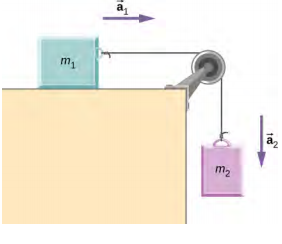1. Shown below are two carts connected by a cord that passes over a small frictionless pulley. Each cart rolls freely with negligible friction. Calculate the acceleration of the carts and the tension in the cord.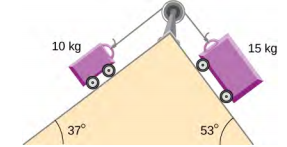1. A 2.00 kg block (mass 1) and a 4.00 kg block (mass 2) are connected by a light string as shown; the inclination of the ramp is 40.0°. Friction is negligible. What is (a) the acceleration of each block and (b) the tension in the string?### 6.2 Friction

1. (a) When rebuilding his car’s engine, a physics major must exert 3.00 x 102 N of force to insert a dry steel piston into a steel cylinder. What is the normal force between the piston and cylinder? (b) What force would he have to exert if the steel parts were oiled?
2. (a) What is the maximum frictional force in the knee joint of a person who supports 66.0 kg of her mass on that knee? (b) During strenuous exercise, it is possible to exert forces to the joints that are easily 10 times greater than the weight being supported. What is the maximum force of friction under such conditions? The frictional forces in joints are relatively small in all circumstances except when the joints deteriorate, such as from injury or arthritis. Increased frictional forces can cause further damage and pain.
3. Suppose you have a 120-kg wooden crate resting on a wood floor, with coefficient of static friction 0.500 between these wood surfaces. (a) What maximum force can you exert horizontally on the crate without moving it? (b) If you continue to exert this force once the crate starts to slip, what will its acceleration then be? The coefficient of sliding friction is known to be 0.300 for this situation.
4. (a) If half of the weight of a small 1.00 x 103-kg utility truck is supported by its two drive wheels, what is the maximum acceleration it can achieve on dry concrete? (b) Will a metal cabinet lying on the wooden bed of the truck slip if it accelerates at this rate? (c) Solve both problems assuming the truck has four-wheel drive.
5. A team of eight dogs pulls a sled with waxed wood runners on wet snow (mush!). The dogs have average masses of 19.0 kg, and the loaded sled with its rider has a mass of 210 kg. (a) Calculate the acceleration of the dogs starting from rest if each dog exerts an average force of 185 N backward on the snow. (b) Calculate the force in the coupling between the dogs and the sled.
6. Consider the 65.0-kg ice skater being pushed by two others shown below. (a) Find the direction and magnitude of Ftot, the total force exerted on her by the others, given that the magnitudes F1 and F2 are 26.4 N and 18.6 N, respectively. (b) What is her initial acceleration if she is initially stationary and wearing steel-bladed skates that point in the direction of Ftot? (c) What is her acceleration assuming she is already moving in the direction of Ftot? (Remember that friction always acts in the direction opposite that of motion or attempted motion between surfaces in contact.)1. Show that the acceleration of any object down a frictionless incline that makes an angle $$\theta$$ with the horizontal is a = g sin $$\theta$$. (Note that this acceleration is independent of mass.)1. Show that the acceleration of any object down an incline where friction behaves simply (that is, where fk = $$\mu_{k}$$N) is a = g(sin $$\theta$$ − $$\mu_{k}$$ cos $$\theta$$). Note that the acceleration is independent of mass and reduces to the expression found in the previous problem when friction becomes negligibly small ($$\mu_{k}$$ = 0).1. Calculate the deceleration of a snow boarder going up a 5.00° slope, assuming the coefficient of friction for waxed wood on wet snow. The result of the preceding problem may be useful, but be careful to consider the fact that the snow boarder is going uphill.
2. A machine at a post office sends packages out a chute and down a ramp to be loaded into delivery vehicles. (a) Calculate the acceleration of a box heading down a 10.0° slope, assuming the coefficient of friction for a parcel on waxed wood is 0.100. (b) Find the angle of the slope down which this box could move at a constant velocity. You can neglect air resistance in both parts.
3. If an object is to rest on an incline without slipping, then friction must equal the component of the weight of the object parallel to the incline. This requires greater and greater friction for steeper slopes. Show that the maximum angle of an incline above the horizontal for which an object will not slide down is $$\theta$$ = tan−1 $$\mu_{s}$$. You may use the result of the previous problem. Assume that a = 0 and that static friction has reached its maximum value.1. Calculate the maximum acceleration of a car that is heading down a 6.00° slope (one that makes an angle of 6.00° with the horizontal) under the following road conditions. You may assume that the weight of the car is evenly distributed on all four tires and that the coefficient of static friction is involved—that is, the tires are not allowed to slip during the deceleration. (Ignore rolling.) Calculate for a car: (a) On dry concrete. (b) On wet concrete. (c) On ice, assuming that $$\mu_{s}$$ = 0.100, the same as for shoes on ice.
2. Calculate the maximum acceleration of a car that is heading up a 4.00° slope (one that makes an angle of 4.00° with the horizontal) under the following road conditions. Assume that only half the weight of the car is supported by the two drive wheels and that the coefficient of static friction is involved—that is, the tires are not allowed to slip during the acceleration. (Ignore rolling.) (a) On dry concrete. (b) On wet concrete. (c) On ice, assuming that $$\mu_{s}$$ = 0.100, the same as for shoes on ice.
3. Repeat the preceding problem for a car with four-wheel drive.
4. A freight train consists of two 8.00 x 105-kg engines and 45 cars with average masses of 5.50 x 105 kg. (a) What force must each engine exert backward on the track to accelerate the train at a rate of 5.00 x 10−2 m/s2 if the force of friction is 7.50 x 105 N, assuming the engines exert identical forces? This is not a large frictional force for such a massive system. Rolling friction for trains is small, and consequently, trains are very energy-efficient transportation systems. (b) What is the force in the coupling between the 37th and 38th cars (this is the force each exerts on the other), assuming all cars have the same mass and that friction is evenly distributed among all of the cars and engines?
5. Consider the 52.0-kg mountain climber shown below. (a) Find the tension in the rope and the force that the mountain climber must exert with her feet on the vertical rock face to remain stationary. Assume that the force is exerted parallel to her legs. Also, assume negligible force exerted by her arms. (b) What is the minimum coefficient of friction between her shoes and the cliff?1. A contestant in a winter sporting event pushes a 45.0-kg block of ice across a frozen lake as shown below. (a) Calculate the minimum force F he must exert to get the block moving. (b) What is its acceleration once it starts to move, if that force is maintained?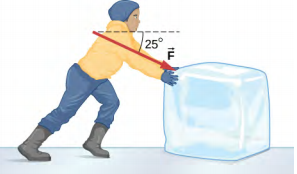1. The contestant now pulls the block of ice with a rope over his shoulder at the same angle above the horizontal as shown below. Calculate the minimum force F he must exert to get the block moving. (b) What is its acceleration once it starts to move, if that force is maintained?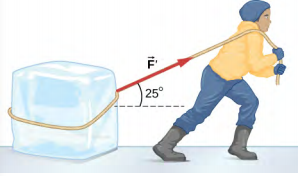1. At a post office, a parcel that is a 20.0-kg box slides down a ramp inclined at 30.0° with the horizontal. The coefficient of kinetic friction between the box and plane is 0.0300. (a) Find the acceleration of the box. (b) Find the velocity of the box as it reaches the end of the plane, if the length of the plane is 2 m and the box starts at rest.

### 6.3 Centripetal Force

1. (a) A 22.0-kg child is riding a playground merry-go-round that is rotating at 40.0 rev/min. What centripetal force is exerted if he is 1.25 m from its center? (b) What centripetal force is exerted if the merry-go-round rotates at 3.00 rev/min and he is 8.00 m from its center? (c) Compare each force with his weight.
2. Calculate the centripetal force on the end of a 100-m (radius) wind turbine blade that is rotating at 0.5 rev/s. Assume the mass is 4 kg.
3. What is the ideal banking angle for a gentle turn of 1.20-km radius on a highway with a 105 km/h speed limit (about 65 mi/h), assuming everyone travels at the limit?
4. What is the ideal speed to take a 100.0-m-radius curve banked at a 20.0° angle?
5. (a) What is the radius of a bobsled turn banked at 75.0° and taken at 30.0 m/s, assuming it is ideally banked? (b) Calculate the centripetal acceleration. (c) Does this acceleration seem large to you?
6. Part of riding a bicycle involves leaning at the correct angle when making a turn, as seen below. To be stable, the force exerted by the ground must be on a line going through the center of gravity. The force on the bicycle wheel can be resolved into two perpendicular components—friction parallel to the road (this must supply the centripetal force) and the vertical normal force (which must equal the system’s weight). (a) Show that $$\theta$$ (as defined as shown) is related to the speed v and radius of curvature r of the turn in the same way as for an ideally banked roadway—that is, $$\theta$$ = tan−1 $$\left(\dfrac{v^{2}}{rg}\right)$$. (b) Calculate $$\theta$$ for a 12.0-m/s turn of radius 30.0 m (as in a race).1. If a car takes a banked curve at less than the ideal speed, friction is needed to keep it from sliding toward the inside of the curve (a problem on icy mountain roads). (a) Calculate the ideal speed to take a 100.0 m radius curve banked at 15.0°. (b) What is the minimum coefficient of friction needed for a frightened driver to take the same curve at 20.0 km/h?
2. Modern roller coasters have vertical loops like the one shown here. The radius of curvature is smaller at the top than on the sides so that the downward centripetal acceleration at the top will be greater than the acceleration due to gravity, keeping the passengers pressed firmly into their seats. What is the speed of the roller coaster at the top of the loop if the radius of curvature there is 15.0 m and the downward acceleration of the car is 1.50 g?1. A child of mass 40.0 kg is in a roller coaster car that travels in a loop of radius 7.00 m. At point A the speed of the car is 10.0 m/s, and at point B, the speed is 10.5 m/s. Assume the child is not holding on and does not wear a seat belt. (a) What is the force of the car seat on the child at point A? (b) What is the force of the car seat on the child at point B? (c) What minimum speed is required to keep the child in his seat at point A?1. In the simple Bohr model of the ground state of the hydrogen atom, the electron travels in a circular orbit around a fixed proton. The radius of the orbit is 5.28 x 10−11 m, and the speed of the electron is 2.18 x 106 m/s. The mass of an electron is 9.11 x 10−31 kg. What is the force on the electron?
2. Railroad tracks follow a circular curve of radius 500.0 m and are banked at an angle of 5.0°. For trains of what speed are these tracks designed?
3. The CERN particle accelerator is circular with a circumference of 7.0 km. (a) What is the acceleration of the protons (m = 1.67 x 10−27 kg) that move around the accelerator at 5% of the speed of light? (The speed of light is v = 3.00 x 108 m/s.) (b) What is the force on the protons?
4. A car rounds an unbanked curve of radius 65 m. If the coefficient of static friction between the road and car is 0.70, what is the maximum speed at which the car traverse the curve without slipping?
5. A banked highway is designed for traffic moving at 90.0 km/h. The radius of the curve is 310 m. What is the angle of banking of the highway?

### 6.4 Drag Force and Terminal Speed

1. The terminal velocity of a person falling in air depends upon the weight and the area of the person facing the fluid. Find the terminal velocity (in meters per second and kilometers per hour) of an 80.0-kg skydiver falling in a pike (headfirst) position with a surface area of 0.140 m2.
2. A 60.0-kg and a 90.0-kg skydiver jump from an airplane at an altitude of 6.00 x 103 m, both falling in the pike position. Make some assumption on their frontal areas and calculate their terminal velocities. How long will it take for each skydiver to reach the ground (assuming the time to reach terminal velocity is small)? Assume all values are accurate to three significant digits.
3. A 560-g squirrel with a surface area of 930 cm2 falls from a 5.0-m tree to the ground. Estimate its terminal velocity. (Use a drag coefficient for a horizontal skydiver.) What will be the velocity of a 56-kg person hitting the ground, assuming no drag contribution in such a short distance?
4. To maintain a constant speed, the force provided by a car’s engine must equal the drag force plus the force of friction of the road (the rolling resistance). (a) What are the drag forces at 70 km/h and 100 km/h for a Toyota Camry? (Drag area is 0.70 m2) (b) What is the drag force at 70 km/h and 100 km/h for a Hummer H2? (Drag area is 2.44 m2) Assume all values are accurate to three significant digits.
5. By what factor does the drag force on a car increase as it goes from 65 to 110 km/h?
6. Calculate the velocity a spherical rain drop would achieve falling from 5.00 km (a) in the absence of air drag (b) with air drag. Take the size across of the drop to be 4 mm, the density to be 1.00 x 103 kg/m3, and the surface area to be $$\pi$$r2.
7. Using Stokes’ law, verify that the units for viscosity are kilograms per meter per second.
8. Find the terminal velocity of a spherical bacterium (diameter 2.00 $$\mu_{m}$$) falling in water. You will first need to note that the drag force is equal to the weight at terminal velocity. Take the density of the bacterium to be 1.10 x 103 kg/m3.
9. Stokes’ law describes sedimentation of particles in liquids and can be used to measure viscosity. Particles in liquids achieve terminal velocity quickly. One can measure the time it takes for a particle to fall a certain distance and then use Stokes’ law to calculate the viscosity of the liquid. Suppose a steel ball bearing (density 7.8 x 103 kg/m3, diameter 3.0 mm) is dropped in a container of motor oil. It takes 12 s to fall a distance of 0.60 m. Calculate the viscosity of the oil.
10. Suppose that the resistive force of the air on a skydiver can be approximated by f = −bv2. If the terminal velocity of a 50.0-kg skydiver is 60.0 m/s, what is the value of b?
11. A small diamond of mass 10.0 g drops from a swimmer’s earring and falls through the water, reaching a terminal velocity of 2.0 m/s. (a) Assuming the frictional force on the diamond obeys f = −bv, what is b? (b) How far does the diamond fall before it reaches 90 percent of its terminal speed?

1. (a) What is the final velocity of a car originally traveling at 50.0 km/h that decelerates at a rate of 0.400 m/s2 for 50.0 s? Assume a coefficient of friction of 1.0. (b) What is unreasonable about the result? (c) Which premise is unreasonable, or which premises are inconsistent?
2. A 75.0-kg woman stands on a bathroom scale in an elevator that accelerates from rest to 30.0 m/s in 2.00 s. (a) Calculate the scale reading in newtons and compare it with her weight. (The scale exerts an upward force on her equal to its reading.) (b) What is unreasonable about the result? (c) Which premise is unreasonable, or which premises are inconsistent?
3. (a) Calculate the minimum coefficient of friction needed for a car to negotiate an unbanked 50.0 m radius curve at 30.0 m/s. (b) What is unreasonable about the result? (c) Which premises are unreasonable or inconsistent?
4. As shown below, if M = 5.50 kg, what is the tension in string 1?1. As shown below, if F = 60.0 N and M = 4.00 kg, what is the magnitude of the acceleration of the suspended object? All surfaces are frictionless.1. As shown below, if M = 6.0 kg, what is the tension in the connecting string? The pulley and all surfaces are frictionless.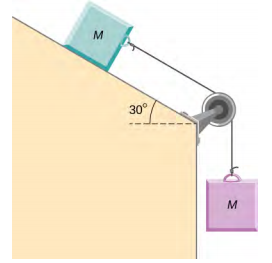1. A small space probe is released from a spaceship. The space probe has mass 20.0 kg and contains 90.0 kg of fuel. It starts from rest in deep space, from the origin of a coordinate system based on the spaceship, and burns fuel at the rate of 3.00 kg/s. The engine provides a constant thrust of 120.0 N. (a) Write an expression for the mass of the space probe as a function of time, between 0 and 30 seconds, assuming that the engine ignites fuel beginning at t = 0. (b) What is the velocity after 15.0 s? (c) What is the position of the space probe after 15.0 s, with initial position at the origin? (d) Write an expression for the position as a function of time, for t > 30.0 s.
2. A half-full recycling bin has mass 3.0 kg and is pushed up a 40.0° incline with constant speed under the action of a 26-N force acting up and parallel to the incline. The incline has friction. What magnitude force must act up and parallel to the incline for the bin to move down the incline at constant velocity?
3. A child has mass 6.0 kg and slides down a 35° incline with constant speed under the action of a 34-N force acting up and parallel to the incline. What is the coefficient of kinetic friction between the child and the surface of the incline?
4. The two barges shown here are coupled by a cable of negligible mass. The mass of the front barge is 2.00 x 103 kg and the mass of the rear barge is 3.00 x 103 kg. A tugboat pulls the front barge with a horizontal force of magnitude 20.0 x 103 N, and the frictional forces of the water on the front and rear barges are 8.00 x 103 N and 10.0 x 103 N, respectively. Find the horizontal acceleration of the barges and the tension in the connecting cable.1. If the order of the barges of the preceding exercise is reversed so that the tugboat pulls the 3.00 x 103 -kg barge with a force of 20.0 x 103 N, what are the acceleration of the barges and the tension in the coupling cable?
2. An object with mass m moves along the x-axis. Its position at any time is given by x(t) = pt3 + qt2 where p and q are constants. Find the net force on this object for any time t.
3. A helicopter with mass 2.35 x 104 kg has a position given by $$\vec{r}$$(t) = (0.020 t3) $$\hat{i}$$ + (2.2t) $$\hat{j}$$ − (0.060 t2) |9\hat{k}\). Find the net force on the helicopter at t = 3.0 s.
4. Located at the origin, an electric car of mass m is at rest and in equilibrium. A time dependent force of $$\vec{F}$$(t) is applied at time t = 0, and its components are Fx(t) = p + nt and Fy(t) = qt where p, q, and n are constants. Find the position $$\vec{r}$$(t) and velocity $$\vec{v}$$(t) as functions of time t.
5. A particle of mass m is located at the origin. It is at rest and in equilibrium. A time-dependent force of $$\vec{F}$$(t) is applied at time t = 0, and its components are Fx(t) = pt and Fy(t) = n + qt where p, q, and n are constants. Find the position $$\vec{r}$$(t) and velocity $$\vec{v}$$(t) as functions of time t.
6. A 2.0-kg object has a velocity of 4.0 $$\hat{i}$$ m/s at t = 0. A constant resultant force of (2.0 $$\hat{i}$$ + 4.0 $$\hat{j}$$) N then acts on the object for 3.0 s. What is the magnitude of the object’s velocity at the end of the 3.0-s interval?
7. A 1.5-kg mass has an acceleration of (4.0 $$\hat{i}$$ − 3.0 $$\hat{j}$$) m/s2. Only two forces act on the mass. If one of the forces is (2.0 $$\hat{i}$$ − 1.4 $$\hat{j}$$) N, what is the magnitude of the other force?
8. A box is dropped onto a conveyor belt moving at 3.4 m/s. If the coefficient of friction between the box and the belt is 0.27, how long will it take before the box moves without slipping?
9. Shown below is a 10.0-kg block being pushed by a horizontal force $$\vec{F}$$ of magnitude 200.0 N. The coefficient of kinetic friction between the two surfaces is 0.50. Find the acceleration of the block.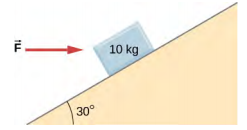1. As shown below, the mass of block 1 is m1 = 4.0 kg, while the mass of block 2 is m2 = 8.0 kg. The coefficient of friction between m1 and the inclined surface is $$\mu_{k}$$ = 0.40. What is the acceleration of the system?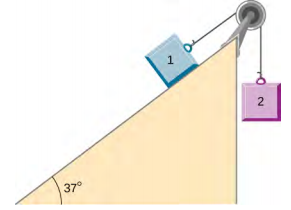1. A student is attempting to move a 30-kg mini-fridge into her dorm room. During a moment of inattention, the mini-fridge slides down a 35 degree incline at constant speed when she applies a force of 25 N acting up and parallel to the incline. What is the coefficient of kinetic friction between the fridge and the surface of the incline?
2. A crate of mass 100.0 kg rests on a rough surface inclined at an angle of 37.0° with the horizontal. A massless rope to which a force can be applied parallel to the surface is attached to the crate and leads to the top of the incline. In its present state, the crate is just ready to slip and start to move down the plane. The coefficient of friction is 80% of that for the static case. (a) What is the coefficient of static friction? (b) What is the maximum force that can be applied upward along the plane on the rope and not move the block? (c) With a slightly greater applied force, the block will slide up the plane. Once it begins to move, what is its acceleration and what reduced force is necessary to keep it moving upward at constant speed? (d) If the block is given a slight nudge to get it started down the plane, what will be its acceleration in that direction? (e) Once the block begins to slide downward, what upward force on the rope is required to keep the block from accelerating downward?
3. A car is moving at high speed along a highway when the driver makes an emergency braking. The wheels become locked (stop rolling), and the resulting skid marks are 32.0 meters long. If the coefficient of kinetic friction between tires and road is 0.550, and the acceleration was constant during braking, how fast was the car going when the wheels became locked?
4. A crate having mass 50.0 kg falls horizontally off the back of the flatbed truck, which is traveling at 100 km/h. Find the value of the coefficient of kinetic friction between the road and crate if the crate slides 50 m on the road in coming to rest. The initial speed of the crate is the same as the truck, 100 km/h.1. A 15-kg sled is pulled across a horizontal, snow-covered surface by a force applied to a rope at 30 degrees with the horizontal. The coefficient of kinetic friction between the sled and the snow is 0.20. (a) If the force is 33 N, what is the horizontal acceleration of the sled? (b) What must the force be in order to pull the sled at constant velocity?
2. A 30.0-g ball at the end of a string is swung in a vertical circle with a radius of 25.0 cm. The tangential velocity is 200.0 cm/s. Find the tension in the string: (a) at the top of the circle, (b) at the bottom of the circle, and (c) at a distance of 12.5 cm from the center of the circle (r = 12.5 cm).
3. A particle of mass 0.50 kg starts moves through a circular path in the xy-plane with a position given by $$\vec{r}$$(t) = (4.0 cos 3t) $$\hat{i}$$ + (4.0 sin 3t) $$\hat{j}$$ where r is in meters and t is in seconds. (a) Find the velocity and acceleration vectors as functions of time. (b) Show that the acceleration vector always points toward the center of the circle (and thus represents centripetal acceleration). (c) Find the centripetal force vector as a function of time.
4. A stunt cyclist rides on the interior of a cylinder 12 m in radius. The coefficient of static friction between the tires and the wall is 0.68. Find the value of the minimum speed for the cyclist to perform the stunt.
5. When a body of mass 0.25 kg is attached to a vertical massless spring, it is extended 5.0 cm from its unstretched length of 4.0 cm. The body and spring are placed on a horizontal frictionless surface and rotated about the held end of the spring at 2.0 rev/s. How far is the spring stretched?
6. Railroad tracks follow a circular curve of radius 500.0 m and are banked at an angle of 5.00°. For trains of what speed are these tracks designed?
7. A plumb bob hangs from the roof of a railroad car. The car rounds a circular track of radius 300.0 m at a speed of 90.0 km/h. At what angle relative to the vertical does the plumb bob hang?
8. An airplane flies at 120.0 m/s and banks at a 30° angle. If its mass is 2.50 x 103 kg, (a) what is the magnitude of the lift force? (b) what is the radius of the turn?
9. The position of a particle is given by $$\vec{r}$$(t) = A (cos $$\omega$$t $$\hat{i}$$ + sin $$\omega$$t $$\hat{j}$$), where $$\omega$$ is a constant. (a) Show that the particle moves in a circle of radius A. (b) Calculate $$\frac{d \vec{r}}{dt}$$ and then show that the speed of the particle is a constant A$$\omega$$. (c) Determine $$\frac{d^{2} \vec{r}}{dt^{2}}$$ and show that a is given by ac = r$$\omega^{2}$$. (d) Calculate the centripetal force on the particle. [Hint: For (b) and (c), you will need to use $$\left(\dfrac{d}{dt}\right)$$(cos $$\omega$$t) = −$$\omega$$ sin $$\omega$$t and $$\left(\dfrac{d}{dt}\right)$$(sin $$\omega$$t) = $$\omega$$ cos $$\omega$$t.
10. Two blocks connected by a string are pulled across a horizontal surface by a force applied to one of the blocks, as shown below. The coefficient of kinetic friction between the blocks and the surface is 0.25. If each block has an acceleration of 2.0 m/s2 to the right, what is the magnitude F of the applied force?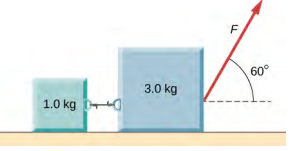1. As shown below, the coefficient of kinetic friction between the surface and the larger block is 0.20, and the coefficient of kinetic friction between the surface and the smaller block is 0.30. If F = 10 N and M = 1.0 kg, what is the tension in the connecting string?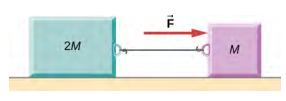1. In the figure, the coefficient of kinetic friction between the surface and the blocks is $$\mu_{k}$$. If M = 1.0 kg, find an expression for the magnitude of the acceleration of either block (in terms of F, $$\mu_{k}$$, and g).1. Two blocks are stacked as shown below, and rest on a frictionless surface. There is friction between the two blocks (coefficient of friction $$\mu$$). An external force is applied to the top block at an angle $$\theta$$ with the horizontal. What is the maximum force F that can be applied for the two blocks to move together?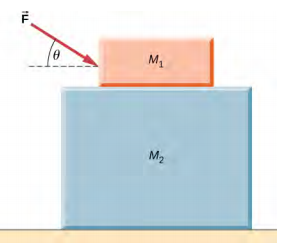1. A box rests on the (horizontal) back of a truck. The coefficient of static friction between the box and the surface on which it rests is 0.24. What maximum distance can the truck travel (starting from rest and moving horizontally with constant acceleration) in 3.0 s without having the box slide?
2. A double-incline plane is shown below. The coefficient of friction on the left surface is 0.30, and on the right surface 0.16. Calculate the acceleration of the system.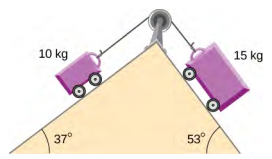## Challenge Problems

1. In a later chapter, you will find that the weight of a particle varies with altitude such that w = $$\frac{mgr_{0}^{2}}{r^{2}}$$ where r0 is the radius of Earth and r is the distance from Earth’s center. If the particle is fired vertically with velocity v0 from Earth’s surface, determine its velocity as a function of position r. (Hint: use a dr = v dv, the rearrangement mentioned in the text.)
2. A large centrifuge, like the one shown below, is used to expose aspiring astronauts to accelerations similar to those experienced in rocket launches and atmospheric reentries. (a) At what angular velocity is the centripetal acceleration 10g if the rider is 15.0 m from the center of rotation? (b) The rider’s cage hangs on a pivot at the end of the arm, allowing it to swing outward during rotation as shown in the bottom accompanying figure. At what angle $$\theta$$ below the horizontal will the cage hang when the centripetal acceleration is 10g? (Hint: The arm supplies centripetal force and supports the weight of the cage. Draw a free-body diagram of the forces to see what the angle $$\theta$$ should be.)1. A car of mass 1000.0 kg is traveling along a level road at 100.0 km/h when its brakes are applied. Calculate the stopping distance if the coefficient of kinetic friction of the tires is 0.500. Neglect air resistance. (Hint: since the distance traveled is of interest rather than the time, x is the desired independent variable and not t. Use the Chain Rule to change the variable: $$\frac{dv}{dt} = \frac{dv}{dx} \frac{dx}{dt} = v \frac{dv}{dx}$$.)
2. An airplane flying at 200.0 m/s makes a turn that takes 4.0 min. What bank angle is required? What is the percentage increase in the perceived weight of the passengers?
3. A skydiver is at an altitude of 1520 m. After 10.0 seconds of free fall, he opens his parachute and finds that the air resistance, FD, is given by the formula FD = −bv, where b is a constant and v is the velocity. If b = 0.750, and the mass of the skydiver is 82.0 kg, first set up differential equations for the velocity and the position, and then find: (a) the speed of the skydiver when the parachute opens, (b) the distance fallen before the parachute opens, (c) the terminal velocity after the parachute opens (find the limiting velocity), and (d) the time the skydiver is in the air after the parachute opens.
4. In a television commercial, a small, spherical bead of mass 4.00 g is released from rest at t = 0 in a bottle of liquid shampoo. The terminal speed is observed to be 2.00 cm/s. Find (a) the value of the constant b in the equation v = $$\frac{mg}{b} \big( 1 − e^{− \frac{bt}{m}} \big)$$, and (b) the value of the resistive force when the bead reaches terminal speed.
5. A boater and motor boat are at rest on a lake. Together, they have mass 200.0 kg. If the thrust of the motor is a constant force of 40.0 N in the direction of motion, and if the resistive force of the water is numerically equivalent to 2 times the speed v of the boat, set up and solve the differential equation to find: (a) the velocity of the boat at time t; (b) the limiting velocity (the velocity after a long time has passed).